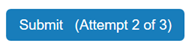## Solve Problems

After signing in to MATLAB® Grader™ and selecting a course, you can find the assignments for the course in the menu on the left. Each assignment in a course contains one or more problems. To complete an assignment, you submit one or more correct solutions for each problem.

Before you submit your solution, you have the option of running the code to check your output ( or ) and to run to run the assessments the instructor has designed for a particular problem.

### Solution Evaluation and Size

After you submit a solution, MATLAB Grader evaluates each solution against the assessment tests that your instructor created. MATLAB Grader determines whether your solution passes some or all of these tests. If your solution passes all of the tests for this problem, MATLAB Grader marks the solution as correct. If your solution fails one or more tests, it marks the solution as incorrect.

#### Solution Size

When you submit a solution, MATLAB Grader determines its size. MATLAB Grader uses a node-count calculation to determine the solution size based on the number of nodes in the parse tree. Think of size as code length. Writing succinct code earns you a better result.

The size of a solution has no effect on the solution score. You can, however, use the size to compare solutions and determine which solution is the best one.

#### Submission Limit

Your instructor may choose to limit the number of solutions you can submit for an assignment. If there is a submission limit, it is indicated on the solve form on the submit button, as "(Attempt X of Y)".When there is only one submission left, you are asked to confirm the final submission. The button becomes disabled after you have submitted the total allowed.

If the instructor has specified that learners may have unlimited submissions, you may submit as many solutions as you want.

### Create a Solution

To create a solution for a problem:

1. Select the problem you want to solve from the list on the Assignment main page or from the menu on the left.

2. Review the description of the problem.

3. Enter your code in the Solution box. Solutions can include MATLAB functions or scripts, depending on your instructor’s requirements. Use or to examine the results of your solution.

4. Test your solution before submitting it by clicking Run Pretests. Note that the option to only appears if your instructor has created pretests for a particular problem.

MATLAB Grader uses the assessment tests for that problem to evaluate your solution. Check the test results to see which test cases passed or failed. Each failed test includes an error message that indicates what caused the test to fail. Tests that passed have a green check mark.5. When you are satisfied with your solution, click Submit. Submitting a solution allows your instructor to see it and the results of any tests for this problem. Only you and your instructor can see your submitted solutions.

#### Late Solutions

You can continue to submit solutions to problems after the assignment due date. However, you cannot be sure that the instructor is going to consider the late solutions when grading your submissions.

#### Example

Assume that a problem in one of your assignments instructs you to use a MATLAB function to find the sum of two numbers. You can read about the plus function in the MATLAB documentation. Using the template in the problem as your starting point, create and test this function in the Solution field:

```function c = add_two_numbers(a,b) %Function for Assignment 1, Problem 1 % Using plus function to add 2 numbers c = plus(a,b); end```

After running your function to verify that it creates the correct output, click Pretests. MATLAB Grader uses the assessment tests to evaluate your solution and shows whether it passed all of the tests cases.

After you have a solution that passes all assessments, click Submit. After you submit the solution, your instructor can view your code and see the results of the assessments for this problem.